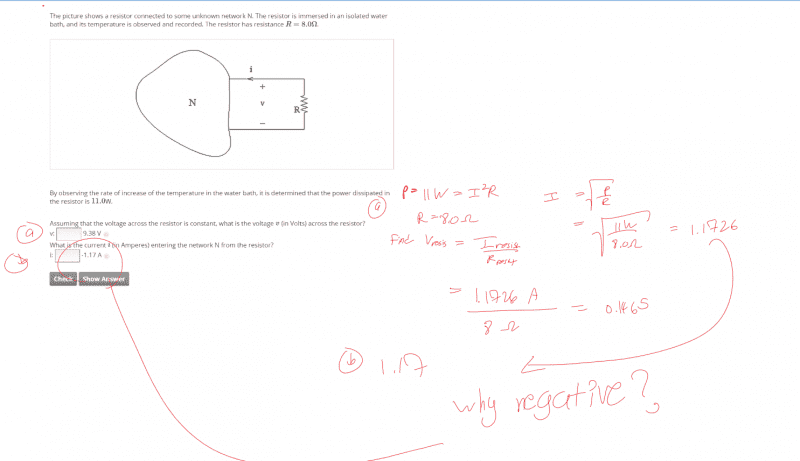# Determining voltage across resistor and current direction

LongApple

## Homework Statement

Resistor conected to unknown network N. Resistor immersed in isolated water bath. Resistor resistance R = 8 ohms. It is determined the power dissipated in resistor is 11 W

a) Find the voltage across the resistor.

b) What is the current i entering the network N from the resistor?
[/B]V=IR

## The Attempt at a Solution

Ignore part a). I understand my mistake for that one.

For part b) though I am trying to get a better intuition for what in this problem would tell us that current is actually leaving the network as opposed to entering it. What is the physical intuition for why that is the case and what is the matematical reason part b) has a negative -1.17 A?